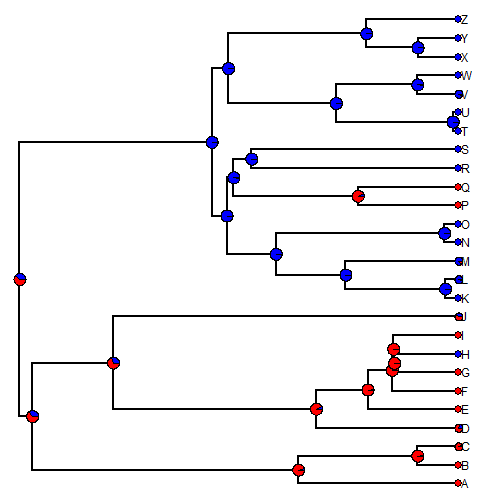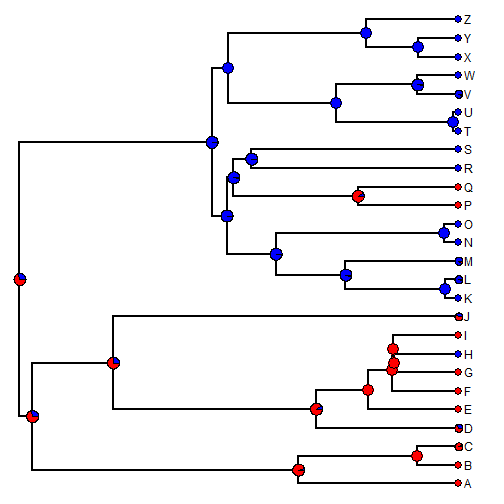## Tuesday, September 1, 2015

### Primer on estimating ancestral states (& tip values) when some tips are unknown

A couple of days ago I received the following question:

“Given a phylogeny and a single binary trait with missing values for some species, I want to predict the value of the missing species. How might you approach this? Thanks!”

Indeed, there are functions in phytools that will predict tip states for both continuous & discretely valued character data. Here is a quick primer.

First, for continuous characters. In this case, the estimates will just be the interpolated states along the edges for the missing tips (since the expected change under BM is zero):

``````## simulate tree & data
x<-fastBM(tree<-pbtree(n=26,tip.label=LETTERS,scale=1))
## simulate missing data
x.sampled<-sample(x,20)
x.sampled
``````
``````##           A           R           K           E           L           Y
##  0.09978808  0.04495263 -0.46726255  1.50418672 -0.43260754 -1.45159378
##           B           T           V           N           O           C
##  1.81665795  0.91084994  0.85232811 -0.13158690 -0.24637438  1.07818611
##           S           Z           G           W           F           J
## -1.09168692 -1.57777654 -0.16152377  1.10174020  0.79768254  1.08410617
##           X           H
## -1.83383452  1.69266010
``````
``````## estimate ancestral states for missing data.
fit<-anc.ML(tree,x.sampled)
fit
``````
``````## \$sig2
##
## 0.6468956
##
## \$ace
##         27         28         29         30         31         32
##  0.4417079  0.4843495  0.7462708  1.3442513  0.6777013  0.9243309
##         33         34         35         36         37         38
##  0.9868763  0.8782826  0.8732871  0.8705345 -0.2067894 -0.2468859
##         39         40         41         42         43         44
## -0.2728397 -0.3430832 -0.4432120 -0.1923593 -0.2617900 -0.2617974
##         45         46         47         48         49         50
## -0.3003078 -0.2182230  0.5498239  0.8936666  0.8909877 -1.2984536
##         51
## -1.5445927
##
## \$logLik
##  -4.705934
##
## \$counts
##      155      155
##
## \$convergence
##  0
##
## \$message
##  "CONVERGENCE: REL_REDUCTION_OF_F <= FACTR*EPSMCH"
##
## \$model
##  "BM"
##
## \$missing.x
##          D          I          M          P          Q          U
##  0.9243847  0.8733192 -0.3431066 -0.2617996 -0.2617996  0.8936641
##
## attr(,"class")
##  "anc.ML"
``````

Now, for discrete characters. In this case, a binary trait:

``````## simulate some data
Q<-matrix(c(-1,1,1,-1),2,2)
rownames(Q)<-colnames(Q)<-0:1
y<-as.factor(sim.history(tree,Q)\$states)
``````
``````## Done simulation(s).
``````
``````y.sampled<-sample(y,20)
y.sampled
``````
``````## K W A T Q U Z H O B F G X P S Y N I R E
## 0 0 1 0 1 0 0 0 0 1 1 1 0 1 0 0 0 1 0 1
## Levels: 0 1
``````
``````## now create a matrix with flat prior probabilities for
## unknown tips
Pr<-to.matrix(y.sampled,seq=levels(y.sampled))
tips<-setdiff(tree\$tip.label,rownames(Pr)) ## missing tips
tips
``````
``````##  "C" "D" "J" "L" "M" "V"
``````
``````Pr<-rbind(Pr,matrix(1/length(levels(y.sampled)),
length(tips),2,dimnames=list(tips)))
Pr
``````
``````##     0   1
## K 1.0 0.0
## W 1.0 0.0
## A 0.0 1.0
## T 1.0 0.0
## Q 0.0 1.0
## U 1.0 0.0
## Z 1.0 0.0
## H 1.0 0.0
## O 1.0 0.0
## B 0.0 1.0
## F 0.0 1.0
## G 0.0 1.0
## X 1.0 0.0
## P 0.0 1.0
## S 1.0 0.0
## Y 1.0 0.0
## N 1.0 0.0
## I 0.0 1.0
## R 1.0 0.0
## E 0.0 1.0
## C 0.5 0.5
## D 0.5 0.5
## J 0.5 0.5
## L 0.5 0.5
## M 0.5 0.5
## V 0.5 0.5
``````
``````fit<-rerootingMethod(tree,Pr,model="ER")
plotTree(tree)
nodelabels(pie=fit\$marginal.anc[as.character(1:tree\$Nnode+Ntip(tree)),],
piecol=c("blue","red"),cex=0.6)
tiplabels(pie=fit\$marginal.anc[tree\$tip.label,],piecol=c("blue","red"),
cex=0.4)
``````This only works for symmetrical models. We can also use `make.simmap` for symmetrical or assymetrical transition matrices:

``````trees<-make.simmap(tree,Pr,model="ARD",nsim=100)
``````
``````## make.simmap is sampling character histories conditioned on the transition matrix
## Q =
``````
``````##            0          1
## 0 -0.4833617  0.4833617
## 1  0.6311203 -0.6311203
``````
``````## (estimated using likelihood);
## and (mean) root node prior probabilities
## pi =
``````
``````##   0   1
## 0.5 0.5
``````
``````## Done.
``````
``````obj<-summary(trees)
plot(obj,colors=setNames(c("blue","red"),levels(y.sampled)))
``````That's it.